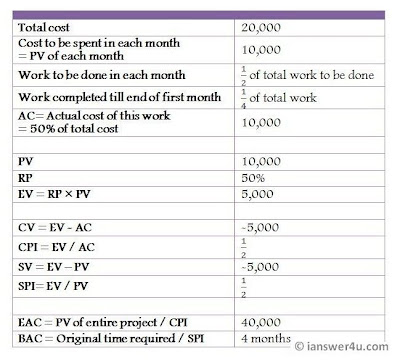# Earned Value Analysis terms and Formulae

Earned Value Management is one of the most common techniques used to measure the performance of the ongoing project. To implement earned value analysis in project management, it is important to first understand the following EVA terms and formulae.

Planned Value (PV):
Also called Budgeted Cost of Work Scheduled(BCWS), is the cost that is proposed to be utilised on an activity during a specific time frame.

Actual Cost (AC):
Also called Actual Cost of Work Performed(ACWP), is the aggregate cost that is spent on an activity while its execution, during a specific time frame.

Rate of Performance (RP):
It is the rate at which the project is progressing. Mathematically, it is the percentage of the work actually completed out of the total work that was scheduled to be completed till that point of time.

Earned Value (EV):
Also called Budgeted Cost of Work Performed(BCWP), is the estimate of the value of the work completed, on the basis of how much work should have been completed and how much work was actually completed.
EV = PV X RPEVA Chart

Illustration to explain these terms:
Consider a project where the work has to be completed in two months in Rs 20,000. Cost breakdown is Rs.10,000 for each month. The work scheduled in each month is half of the total work to be completed.
By the end of the first month, the project has completed only 25% of the total work to be completed, but the cost utilised is that of 50% of the total work.

Also given that, for completion of 25% work, the actual cost(AC) incurred =50% of total budgeted cost
=10,000

Now from given data :-
The planned value(PV) for the work to be completed by the end of the first month
=50% of total
=50% of 20,000
=10,000

The rate of performance(RP)= Percentage of ratio of work actually completed to the work scheduled to be completed
=(25/50)X 100
=50%
This implies that only half of the work scheduled to be completed by the end of the first month has been completed.

Now, the earned value(EV) or the value of the work actually completed in monetary terms
=Rate of performance X Planned Value of work to be completed till the first month (EV = PV X RP)
=50% of 10,000
=5,000
This implies that we have utilised Rs.10,000(AC) for the work which should have utilised Rs. 5,000(EV) according to our budget.

Some more important terms in Earned Value Management:

Cost Variance (CV):
As the name suggests, Cost Variance calculates the deviation between the cost actually incurred and the planned cost. It checks whether we have gone according to our budgeted costs or not.
CV = EV - AC

Cost Performance Index(CPI):
Cost Performance is used to estimate the projected or actual cost of completing the project based on the performance to date.
CPI = EV / AC

Schedule Variance (SV):
Schedule Variance calculates the deviation between the actual and planned time taken to complete the project or an activity of a project. It checks whether the project has taken more or less time than that of the planned schedule.
SV = EV - PV

Schedule Performance Index(SPI):
Schedule Performance Index is used to estimated the projected time to complete the project.
SPI = EV / PV

Estimate At Completion(EAC):
Used to find the estimate cost of the project at completion, going by the present performance.
EAC = PV of whole project/CPI

Estimated time to complete:
Used to find the approximate time required to complete the project, going by the present performance.
=Original time/SPI

Illustration:
Take the above example to understand these terms.

Cost variance(CV)=EV - AC
=5,000-10,000
=-5,000

Cost performance index(CPI)=EV / AC
=5,000/10,000
=1/2

Schedule variance(SV)=EV - PV
=5,000-10,000
=-5,000

Schedule performance index(SPI)=EV / PV
=5,000/10,000
=1/2

Estimate At Completion(EAC)=PV of whole project/CPI
=20,000/(1/2)
=40,000
This indicates that the project which had to be completed in a budget of Rs.20,000 will be completed in Rs.40,000(if the rate of performance is the same).

Estimated time to complete:
=Original time/SPI
=2 months/(1/2)
=4 months
This indicates that the project which had to be completed in 2 months will be completed in 4 months, if the work goes on in the same way.Earned Value Illustration

Negative values denote:
If CV is negative, it means that actual cost of performing the work is more than planned (AC > PV)
If SV is negative, it means that the activity took more time than planned to complete.

CPI interpretations:
=1: the actual cost = planned cost
<1: actual costs are more than the budget >1: actual costs are under budget

SPI interpretations:
=1: on schedule
<1: behind schedule >1: ahead of schedule

After going through the definition, need and terms related to earned value management, I hope you will be able to use this tool properly to rate the performance of your project. Will Earned Value Analysis work for your project?

1.